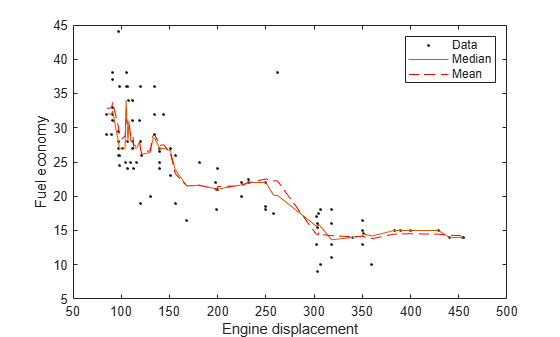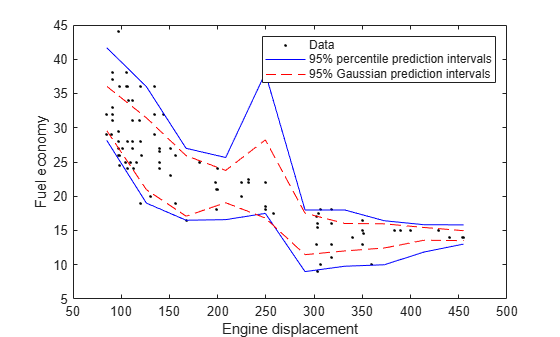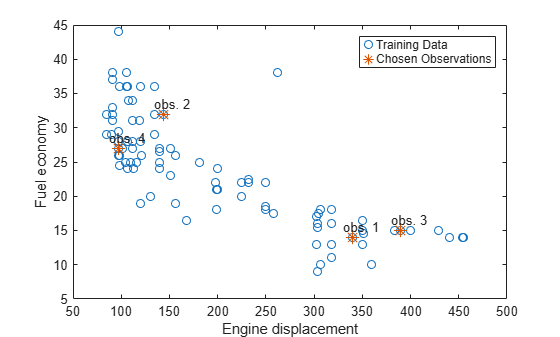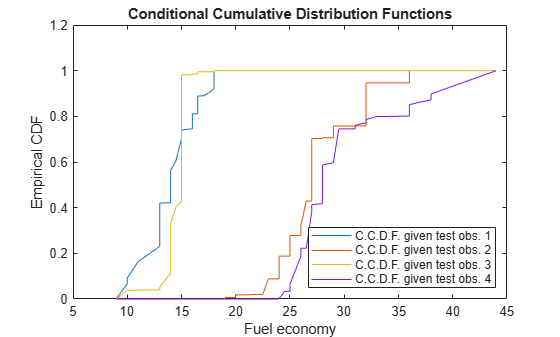quantilePredict

Class: TreeBagger

Predict response quantile using bag of regression trees

Description

example

YFit = quantilePredict(Mdl,X) returns a vector of medians of the predicted responses at X, a table or matrix of predictor data, and using the bag of regression trees Mdl. Mdl must be a TreeBagger model object.

example

YFit = quantilePredict(Mdl,X,Name,Value) uses additional options specified by one or more Name,Value pair arguments. For example, specify quantile probabilities or which trees to include for quantile estimation.

example

[YFit,YW] = quantilePredict(___) also returns a sparse matrix of response weights.

Input Arguments

expand all

Bag of regression trees, specified as a TreeBagger model object created by TreeBagger. The value of Mdl.Method must be regression.

Predictor data used to estimate quantiles, specified as a numeric matrix or table.

Each row of X corresponds to one observation, and each column corresponds to one variable.

• For a numeric matrix:

• The variables making up the columns of X must have the same order as the predictor variables that trained Mdl.

• If you trained Mdl using a table (for example, Tbl), then X can be a numeric matrix if Tbl contains all numeric predictor variables. If Tbl contains heterogeneous predictor variables (for example, numeric and categorical data types) and X is a numeric matrix, then quantilePredict throws an error.

• For a table:

• quantilePredict does not support multicolumn variables and cell arrays other than cell arrays of character vectors.

• If you trained Mdl using a table (for example, Tbl), then all predictor variables in X must have the same variable names and data types as those variables that trained Mdl (stored in Mdl.PredictorNames). However, the column order of X does not need to correspond to the column order of Tbl. Tbl and X can contain additional variables (response variables, observation weights, etc.), but quantilePredict ignores them.

• If you trained Mdl using a numeric matrix, then the predictor names in Mdl.PredictorNames and corresponding predictor variable names in X must be the same. To specify predictor names during training, see the PredictorNames name-value pair argument of TreeBagger. All predictor variables in X must be numeric vectors. X can contain additional variables (response variables, observation weights, etc.), but quantilePredict ignores them.

Data Types: table | double | single

Name-Value Arguments

Specify optional comma-separated pairs of Name,Value arguments. Name is the argument name and Value is the corresponding value. Name must appear inside quotes. You can specify several name and value pair arguments in any order as Name1,Value1,...,NameN,ValueN.

Quantile probability, specified as the comma-separated pair consisting of 'Quantile' and a numeric vector containing values in the interval [0,1]. For each observation (row) in X, quantilePredict returns corresponding quantiles for all probabilities in Quantile.

Example: 'Quantile',[0 0.25 0.5 0.75 1]

Data Types: single | double

Indices of trees to use in response estimation, specified as the comma-separated pair consisting of 'Trees' and 'all' or a numeric vector of positive integers. Indices correspond to the cells of Mdl.Trees; each cell therein contains a tree in the ensemble. The maximum value of Trees must be less than or equal to the number of trees in the ensemble (Mdl.NumTrees).

For 'all', quantilePredict uses the indices 1:Mdl.NumTrees.

Example: 'Trees',[1 10 Mdl.NumTrees]

Data Types: char | string | single | double

Weights to attribute to responses from individual trees, specified as the comma-separated pair consisting of 'TreeWeights' and a numeric vector of numel(trees) nonnegative values. trees is the value of the Trees name-value pair argument.

The default is ones(size(trees)).

Data Types: single | double

Indicators specifying which trees to use to make predictions for each observation, specified as the comma-separated pair consisting of 'UseInstanceForTree' and an n-by-Mdl.Trees logical matrix. n is the number of observations (rows) in X. Rows of UseInstanceForTree correspond to observations and columns correspond to learners in Mdl.Trees. 'all' indicates to use all trees for all observations when estimating the quantiles.

If UseInstanceForTree(j,k) = true, then quantilePredict uses the tree in Mdl.Trees(trees(k)) when it predicts the response for the observation X(j,:).

You can estimate the quantile using the response data in Mdl.Y directly instead of using the predictions from the random forest by specifying a row composed entirely of false values. For example, to estimate the quantile for observation j using the response data, and to use the predictions from the random forest for all other observations, specify this matrix:

UseInstanceForTree = true(size(Mdl.X,2),Mdl.NumTrees);
UseInstanceForTree(j,:) = false(1,Mdl.NumTrees);

Data Types: char | string | logical

Output Arguments

expand all

Estimated quantiles, returned as an n-by-numel(tau) numeric matrix. n is the number of observations in X (size(X,1)) and tau is the value of Quantile. That is, YFit(j,k) is the estimated 100*tau(k)% percentile of the response distribution given X(j,:) and using Mdl.

Response weights, returned as an ntrain-by-n sparse matrix. ntrain is the number of responses in the training data (numel(Mdl.Y)) and n is the number of observations in X (size(X,1)).

quantilePredict predicts quantiles using linear interpolation of the empirical cumulative distribution function (C.D.F.). For a particular observation, you can use its response weights to estimate quantiles using alternative methods, such as approximating the C.D.F. using kernel smoothing.

Note

quantilePredict derives response weights by passing an observation through the trees in the ensemble. If you specify UseInstanceForTree and you compose row j entirely of false values, then YW(:,j) = Mdl.W instead, that is, the observation weights.

Examples

expand all

Load the carsmall data set. Consider a model that predicts the fuel economy of a car given its engine displacement.

Train an ensemble of bagged regression trees using the entire data set. Specify 100 weak learners.

rng(1); % For reproducibility
Mdl = TreeBagger(100,Displacement,MPG,'Method','regression');

Mdl is a TreeBagger ensemble.

Perform quantile regression to predict the median MPG for all sorted training observations.

medianMPG = quantilePredict(Mdl,sort(Displacement));

medianMPG is an n-by-1 numeric vector of medians corresponding to the conditional distribution of the response given the sorted observations in Displacement. n is the number of observations in Displacement.

Plot the observations and the estimated medians on the same figure. Compare the median and mean responses.

meanMPG = predict(Mdl,sort(Displacement));

figure;
plot(Displacement,MPG,'k.');
hold on
plot(sort(Displacement),medianMPG);
plot(sort(Displacement),meanMPG,'r--');
ylabel('Fuel economy');
xlabel('Engine displacement');
legend('Data','Median','Mean');
hold off;Load the carsmall data set. Consider a model that predicts the fuel economy of a car given its engine displacement.

Train an ensemble of bagged regression trees using the entire data set. Specify 100 weak learners.

rng(1); % For reproducibility
Mdl = TreeBagger(100,Displacement,MPG,'Method','regression');

Perform quantile regression to predict the 2.5% and 97.5% percentiles for ten equally-spaced engine displacements between the minimum and maximum in-sample displacement.

predX = linspace(min(Displacement),max(Displacement),10)';
quantPredInts = quantilePredict(Mdl,predX,'Quantile',[0.025,0.975]);

quantPredInts is a 10-by-2 numeric matrix of prediction intervals corresponding to the observations in predX. The first column contains the 2.5% percentiles and the second column contains the 97.5% percentiles.

Plot the observations and the estimated medians on the same figure. Compare the percentile prediction intervals and the 95% prediction intervals assuming the conditional distribution of MPG is Gaussian.

[meanMPG,steMeanMPG] = predict(Mdl,predX);
stndPredInts = meanMPG + [-1 1]*norminv(0.975).*steMeanMPG;

figure;
h1 = plot(Displacement,MPG,'k.');
hold on
h2 = plot(predX,quantPredInts,'b');
h3 = plot(predX,stndPredInts,'r--');
ylabel('Fuel economy');
xlabel('Engine displacement');
legend([h1,h2(1),h3(1)],{'Data','95% percentile prediction intervals',...
'95% Gaussian prediction intervals'});
hold off;Load the carsmall data set. Consider a model that predicts the fuel economy of a car given its engine displacement.

Train an ensemble of bagged regression trees using the entire data set. Specify 100 weak learners.

rng(1); % For reproducibility
Mdl = TreeBagger(100,Displacement,MPG,'Method','regression');

Estimate the response weights for a random sample of four training observations. Plot the training sample and identify the chosen observations.

[predX,idx] = datasample(Mdl.X,4);
[~,YW] = quantilePredict(Mdl,predX);
n = numel(Mdl.Y);

figure;
plot(Mdl.X,Mdl.Y,'o');
hold on
plot(predX,Mdl.Y(idx),'*','MarkerSize',10);
text(predX-10,Mdl.Y(idx)+1.5,{'obs. 1' 'obs. 2' 'obs. 3' 'obs. 4'});
legend('Training Data','Chosen Observations');
xlabel('Engine displacement')
ylabel('Fuel economy')
hold offYW is an n-by-4 sparse matrix containing the response weights. Columns correspond to test observations and rows correspond to responses in the training sample. Response weights are independent of the specified quantile probability.

Estimate the conditional cumulative distribution function (C.C.D.F.) of the responses by:

1. Sorting the responses is ascending order, and then sorting the response weights using the indices induced by sorting the responses.

2. Computing the cumulative sums over each column of the sorted response weights.

[sortY,sortIdx] = sort(Mdl.Y);
cpdf = full(YW(sortIdx,:));
ccdf = cumsum(cpdf);

ccdf(:,j) is the empirical C.C.D.F. of the response given test observation j.

Plot the four empirical C.C.D.F. in the same figure.

figure;
plot(sortY,ccdf);
legend('C.C.D.F. given test obs. 1','C.C.D.F. given test obs. 2',...
'C.C.D.F. given test obs. 3','C.C.D.F. given test obs. 4',...
'Location','SouthEast')
title('Conditional Cumulative Distribution Functions')
xlabel('Fuel economy')
ylabel('Empirical CDF')expand all

Tips

quantilePredict estimates the conditional distribution of the response using the training data every time you call it. To predict many quantiles efficiently, or quantiles for many observations efficiently, you should pass X as a matrix or table of observations and specify all quantiles in a vector using the Quantile name-value pair argument. That is, avoid calling quantilePredict within a loop.

Algorithms

• TreeBagger grows a random forest of regression trees using the training data. Then, to implement quantile random forest, quantilePredict predicts quantiles using the empirical conditional distribution of the response given an observation from the predictor variables. To obtain the empirical conditional distribution of the response:

1. quantilePredict passes all the training observations in Mdl.X through all the trees in the ensemble, and stores the leaf nodes of which the training observations are members.

2. quantilePredict similarly passes each observation in X through all the trees in the ensemble.

3. For each observation in X, quantilePredict:

1. Estimates the conditional distribution of the response by computing response weights for each tree.

2. For observation k in X, aggregates the conditional distributions for the entire ensemble:

$\stackrel{^}{F}\left(y|X={x}_{k}\right)=\sum _{j=1}^{n}\sum _{t=1}^{T}\frac{1}{T}{w}_{tj}\left({x}_{k}\right)I\left\{{Y}_{j}\le y\right\}.$

n is the number of training observations (size(Y,1)) and T is the number of trees in the ensemble (Mdl.NumTrees).

4. For observation k in X, the τ quantile or, equivalently, the 100τ% percentile, is ${Q}_{\tau }\left({x}_{k}\right)=\mathrm{inf}\left\{y:\stackrel{^}{F}\left(y|X={x}_{k}\right)\ge \tau \right\}.$

• This process describes how quantilePredict uses all specified weights.

1. For all training observations j = 1,...,n and all chosen trees t = 1,...,T,

quantilePredict attributes the product vtj = btjwj,obs to training observation j (stored in Mdl.X(j,:) and Mdl.Y(j)). btj is the number of times observation j is in the bootstrap sample for tree t. wj,obs is the observation weight in Mdl.W(j).

2. For each chosen tree, quantilePredict identifies the leaves in which each training observation falls. Let St(xj) be the set of all observations contained in the leaf of tree t of which observation j is a member.

3. For each chosen tree, quantilePredict normalizes all weights within a particular leaf to sum to 1, that is,

${v}_{tj}^{\ast }=\frac{{v}_{tj}}{\sum _{i\in {S}_{t}\left({x}_{j}\right)}{v}_{ti}}.$

4. For each training observation and tree, quantilePredict incorporates tree weights (wt,tree) specified by TreeWeights, that is, w*tj,tree = wt,treevtj*Trees not chosen for prediction have 0 weight.

5. For all test observations k = 1,...,K in X and all chosen trees t = 1,...,TquantilePredict predicts the unique leaves in which the observations fall, and then identifies all training observations within the predicted leaves. quantilePredict attributes the weight utj such that

6. quantilePredict sums the weights over all chosen trees, that is,

${u}_{j}=\sum _{t=1}^{T}{u}_{tj}.$

7. quantilePredict creates response weights by normalizing the weights so that they sum to 1, that is,

${w}_{j}^{\ast }=\frac{{u}_{j}}{{\sum }_{j=1}^{n}{u}_{j}}.$

References

 Breiman, L. "Random Forests." Machine Learning 45, pp. 5–32, 2001.

 Meinshausen, N. “Quantile Regression Forests.” Journal of Machine Learning Research, Vol. 7, 2006, pp. 983–999.